# Intermediate Geometry : How to find the perimeter of a hexagon

## Example Questions

1 2 3 5 Next →

### Example Question #41 : How To Find The Perimeter Of A Hexagon

Find the perimeter of the regular hexagon below: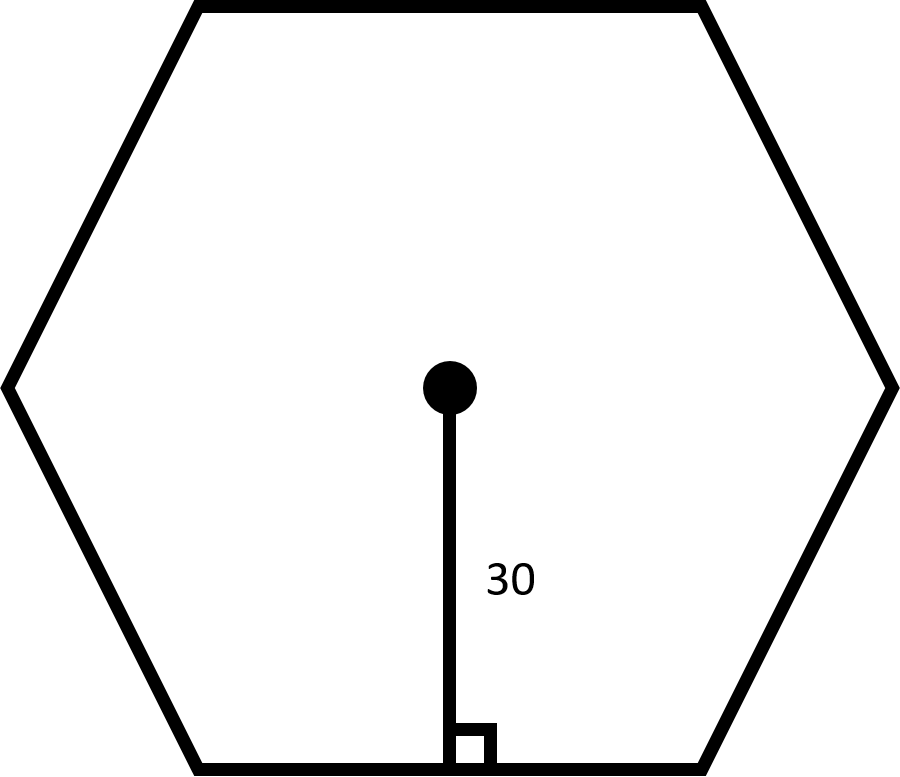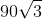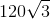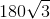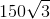Explanation: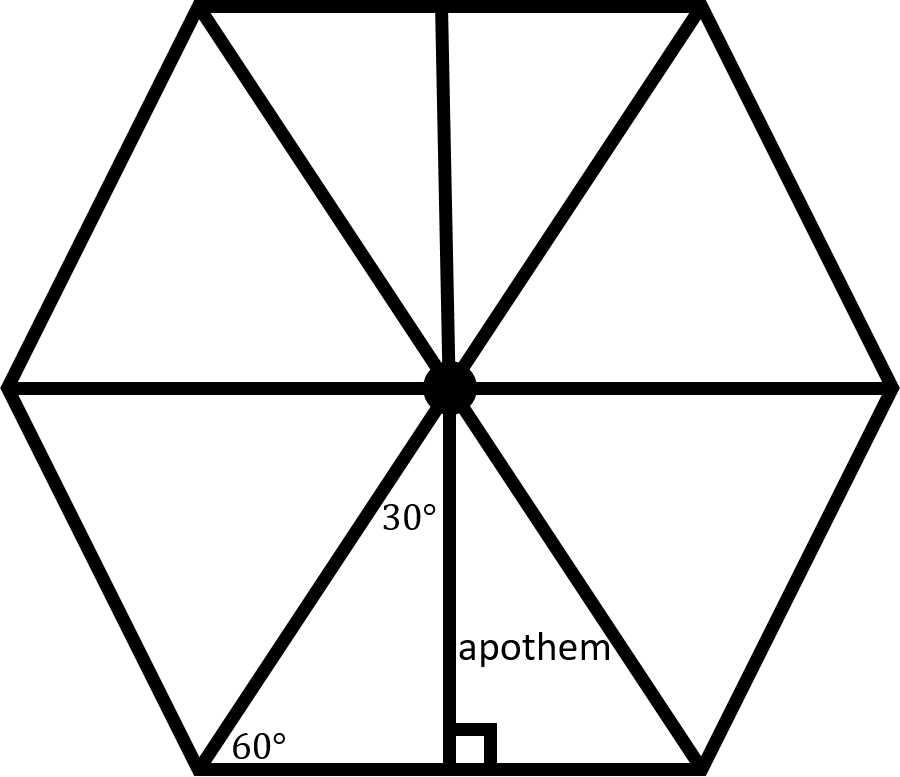When all the diagonals of the regular hexagon are drawn in, you should notice that six congruent equilateral triangles are created.

Thus, we know that the given line segment, also known as an apothem, acts as the height of an equilateral triangle. The apothem also creates two congruent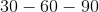triangles.

Thus, we can use the ratio of the side lengths to find the length of the base of one of thetriangles.

Recall that the ratios of the side lengths in atriangle are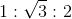.

We can then set up the following equation: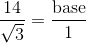The length of the base is then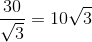.

Now, the length of this base is half the length of a side of the hexagon. Multiply the base by two to find the length of a side: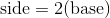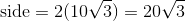Next, recall how to find the perimeter of a regular hexagon: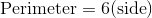Plug in the side length to find the perimeter: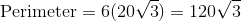### Example Question #42 : How To Find The Perimeter Of A Hexagon

Find the perimeter of the regular hexagon below: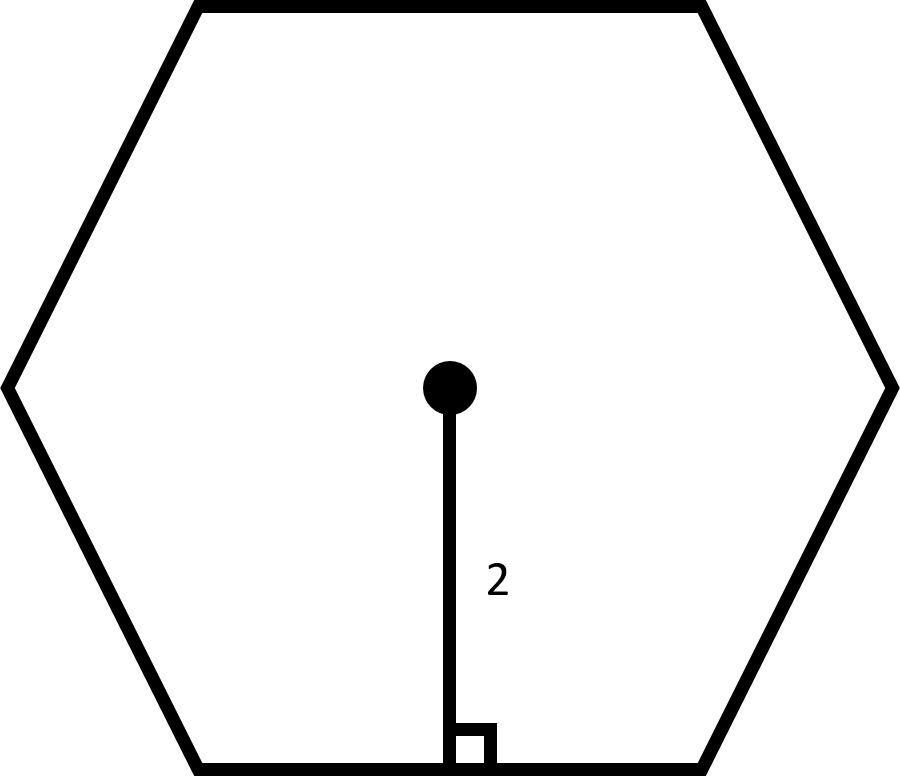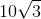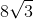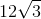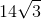Explanation:When all the diagonals of the regular hexagon are drawn in, you should notice that six congruent equilateral triangles are created.

Thus, we know that the given line segment, also known as an apothem, acts as the height of an equilateral triangle. The apothem also creates two congruenttriangles.

Thus, we can use the ratio of the side lengths to find the length of the base of one of thetriangles.

Recall that the ratios of the side lengths in atriangle are.

We can then set up the following equation:The length of the base is then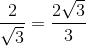.

Now, the length of this base is half the length of a side of the hexagon. Multiply the base by two to find the length of a side: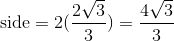Next, recall how to find the perimeter of a regular hexagon:Plug in the side length to find the perimeter: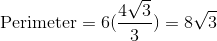### Example Question #43 : How To Find The Perimeter Of A Hexagon

Find the perimeter of the regular hexagon below: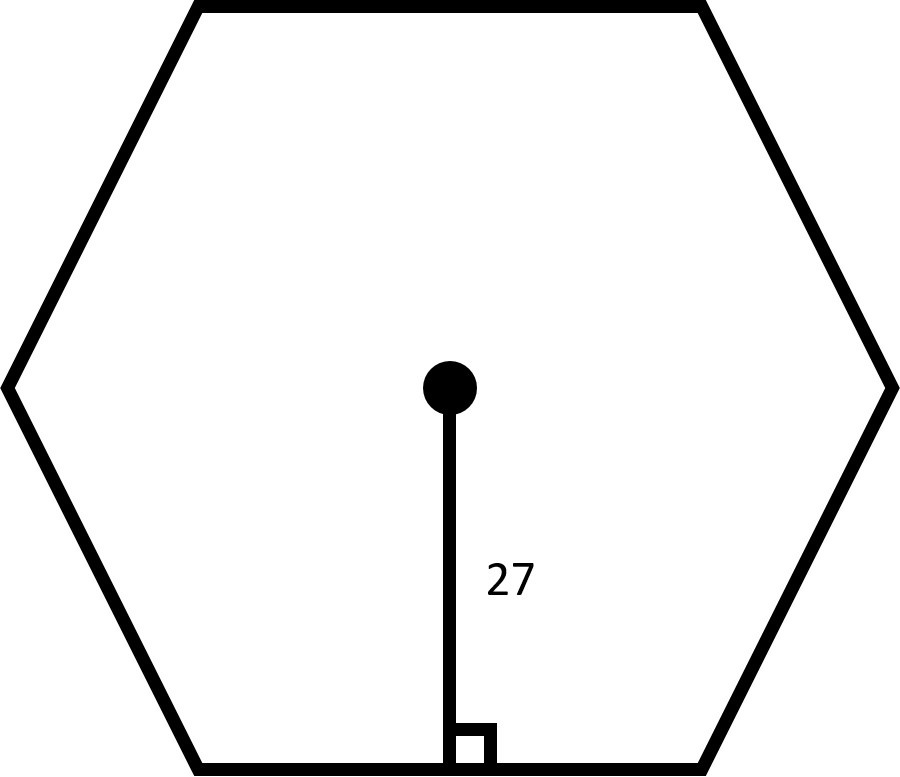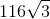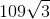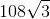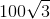Explanation:When all the diagonals of the regular hexagon are drawn in, you should notice that six congruent equilateral triangles are created.

Thus, we know that the given line segment, also known as an apothem, acts as the height of an equilateral triangle. The apothem also creates two congruenttriangles.

Thus, we can use the ratio of the side lengths to find the length of the base of one of thetriangles.

Recall that the ratios of the side lengths in atriangle are.

We can then set up the following equation:The length of the base is then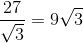.

Now, the length of this base is half the length of a side of the hexagon. Multiply the base by two to find the length of a side: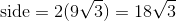Next, recall how to find the perimeter of a regular hexagon:Plug in the side length to find the perimeter: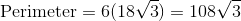### Example Question #44 : How To Find The Perimeter Of A Hexagon

Find the perimeter of the regular hexagon below: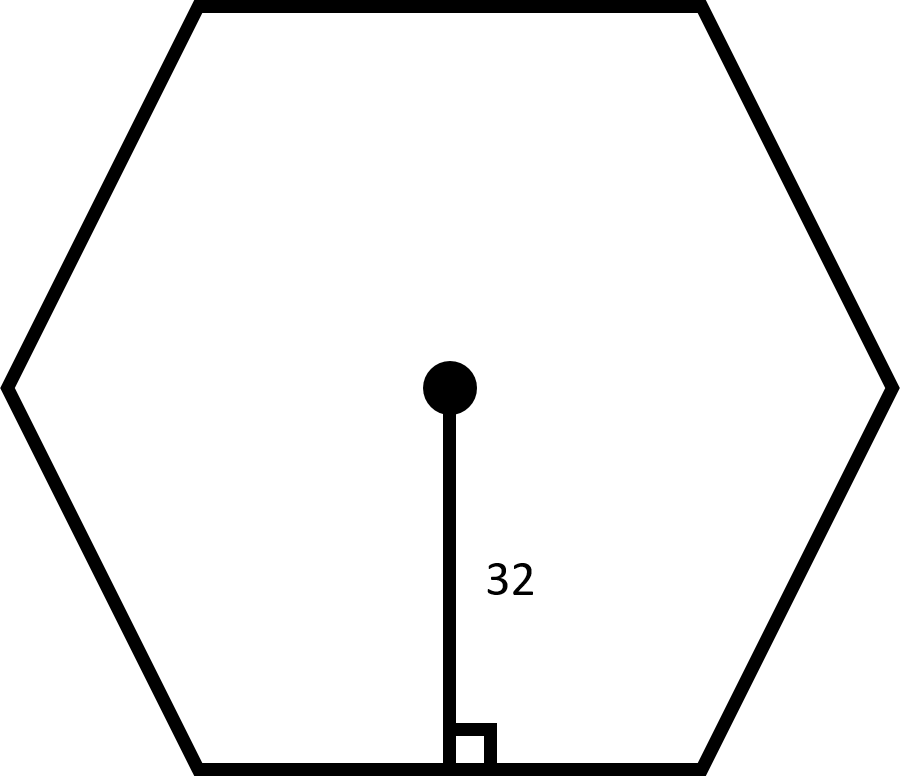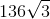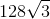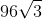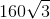Explanation:When all the diagonals of the regular hexagon are drawn in, you should notice that six congruent equilateral triangles are created.

Thus, we know that the given line segment, also known as an apothem, acts as the height of an equilateral triangle. The apothem also creates two congruenttriangles.

Thus, we can use the ratio of the side lengths to find the length of the base of one of thetriangles.

Recall that the ratios of the side lengths in atriangle are.

We can then set up the following equation:The length of the base is then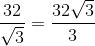.

Now, the length of this base is half the length of a side of the hexagon. Multiply the base by two to find the length of a side: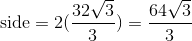Next, recall how to find the perimeter of a regular hexagon:Plug in the side length to find the perimeter: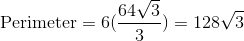### Example Question #41 : How To Find The Perimeter Of A Hexagon

Find the perimeter of the regular hexagon below: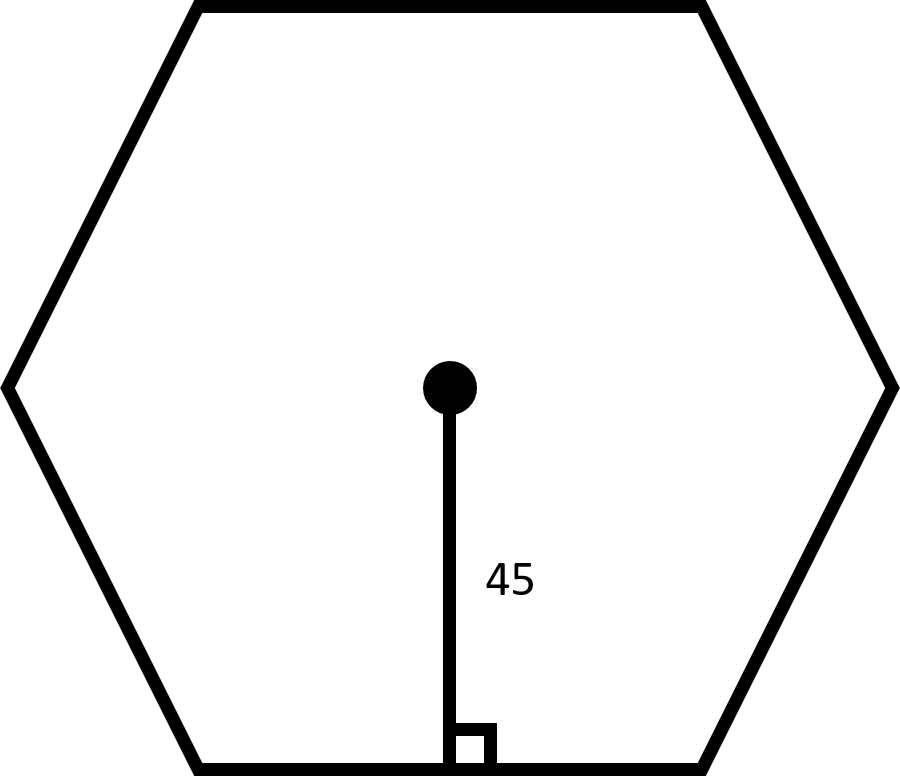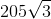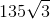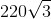Explanation:When all the diagonals of the regular hexagon are drawn in, you should notice that six congruent equilateral triangles are created.

Thus, we know that the given line segment, also known as an apothem, acts as the height of an equilateral triangle. The apothem also creates two congruenttriangles.

Thus, we can use the ratio of the side lengths to find the length of the base of one of thetriangles.

Recall that the ratios of the side lengths in atriangle are.

We can then set up the following equation:The length of the base is then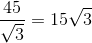.

Now, the length of this base is half the length of a side of the hexagon. Multiply the base by two to find the length of a side: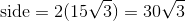Next, recall how to find the perimeter of a regular hexagon:Plug in the side length to find the perimeter: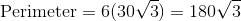1 2 3 5 Next →

### All Intermediate Geometry Resources